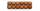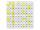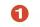# Natural numbers + divisibility - math problems

#### Number of problems found: 122

• Divisibility 2How many divisors has integer number 13?
• DivisibilityDetermine all divisors of number 84.
• Sum of the digitsHow many are two-digit natural numbers that have the sum of the digits 9?
• Twenty-fiveHow many three-digit natural numbers are divisible by 25?
• Three-digit integersHow many three-digit natural numbers exist that do not contain zero and are divisible by five?
• Count of rootsHow many solutions has equation x. y = 7757 with two unknowns on the set of natural numbers?
• Divisible by nineHow many three-digit natural numbers in total are divisible without a remainder by the number 9?
• Primes 2Which prime numbers is number 2025 divisible?
• RectanglesHow many rectangles with area 8713 cm2 whose sides are natural numbers are?
• Year 2018The product of the three positive numbers is 2018. What are the numbers?
• Theorem proveWe want to prove the sentence: If the natural number n is divisible by six, then n is divisible by three. From what assumption we started?
• Seven numbersWrite seven 4-digit numbers that are divisible by 3 and at the same time by 4.
• Common divisorsFind all common divisors of numbers 30 and 45.
• Reminder and quotientThere are given the number C = 281, D = 201. Find the highest natural number S so that the C:S, D:S are with the remainder of 1,
• Sum of odd numbersFind the sum of all odd integers from 13 to 781.
• Odd numbersThe sum of four consecutive odd numbers is 1048. Find those numbers ...
• DivisibilityDetermine the smallest integer which divided 11 gives remainder 4 when divided 15 gives remainder 10 and when divided by 19 gives remainder 16.
• The sumThe sum of five consecutive odd numbers is 75. Find out the sum of the second and fourth of them.
• How manyHow many numbers are less than 222 with a digit sum is 8?
• DivisorsFind all divisors of number 493. How many are them?

Do you have an interesting mathematical word problem that you can't solve it? Submit a math problem, and we can try to solve it.

We will send a solution to your e-mail address. Solved examples are also published here. Please enter the e-mail correctly and check whether you don't have a full mailbox.

Please do not submit problems from current active competitions such as Mathematical Olympiad, correspondence seminars etc...

Natural numbers - math word problems. Divisibility - math word problems.